# Ring theory

In algebra, ring theory is the study of rings—algebraic structures in which addition and multiplication are defined and have similar properties to those operations defined for the integers. Ring theory studies the structure of rings, their representations, or, in different language, modules, special classes of rings (group rings, division rings, universal enveloping algebras), as well as an array of properties that proved to be of interest both within the theory itself and for its applications, such as homological properties and polynomial identities. Commutative rings are much better understood than noncommutative ones. Algebraic geometry and algebraic number theory, which provide many natural examples of commutative rings, have driven much of the development of commutative ring theory, which is now, under the name of commutative algebra, a major area of modern mathematics. Because these three fields (algebraic geometry, algebraic number theory and commutative algebra) are so intimately connected it is usually difficult and meaningless to decide which field a particular result belongs to. For example, Hilbert's Nullstellensatz is a theorem which is fundamental for algebraic geometry, and is stated and proved in terms of commutative algebra. Similarly, Fermat's Last Theorem is stated in terms of elementary arithmetic, which is a part of commutative algebra, but its proof involves deep results of both algebraic number theory and algebraic geometry. Noncommutative rings are quite different in flavour, since more unusual behavior can arise. While the theory has developed in its own right, a fairly recent trend has sought to parallel the commutative development by building the theory of certain classes of noncommutative rings in a geometric fashion as if they were rings of functions on (non-existent) 'noncommutative spaces'. This trend started in the 1980s with the development of noncommutative geometry and with the discovery of quantum groups. It has led to a better understanding of noncommutative rings, especially noncommutative Noetherian rings. For the definitions of a ring and basic concepts and their properties, see Ring (mathematics). The definitions of terms used throughout ring theory may be found in Glossary of ring theory. (Wikipedia).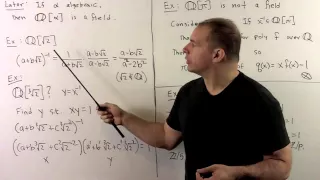RNT1.1. Definition of Ring

Ring Theory: We define rings and give many examples. Items under consideration include commutativity and multiplicative inverses. Example include modular integers, square matrices, polynomial rings, quaternions, and adjoins of algebraic and transcendental numbers.

From playlist Abstract Algebra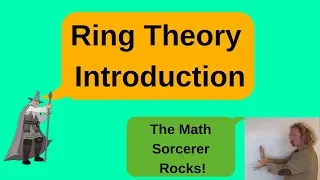Definition of a Ring and Examples of Rings

Please Subscribe here, thank you!!! https://goo.gl/JQ8Nys Definition of a Ring and Examples of Rings - Definition of a Ring. - Definition of a commutative ring and a ring with identity. - Examples of Rings include: Z, Q, R, C under regular addition and multiplication The Ring of all n x

From playlist Abstract Algebra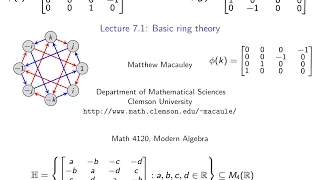Visual Group Theory, Lecture 7.1: Basic ring theory

Visual Group Theory, Lecture 7.1: Basic ring theory A ring is an abelian group (R,+) with a second binary operation, multiplication and the distributive law. Multiplication need not commute, nor need there be multiplicative inverses, so a ring is like a field but without these properties.

From playlist Visual Group Theory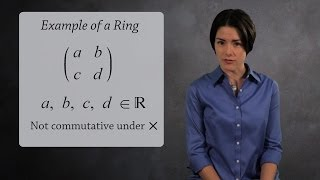Abstract Algebra: The definition of a Ring

Learn the definition of a ring, one of the central objects in abstract algebra. We give several examples to illustrate this concept including matrices and polynomials. Be sure to subscribe so you don't miss new lessons from Socratica: http://bit.ly/1ixuu9W ♦♦♦♦♦♦♦♦♦♦ We recommend th

From playlist Abstract Algebra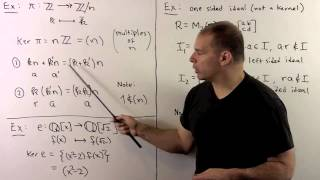RNT1.4. Ideals and Quotient Rings

Ring Theory: We define ideals in rings as an analogue of normal subgroups in group theory. We give a correspondence between (two-sided) ideals and kernels of homomorphisms using quotient rings. We also state the First Isomorphism Theorem for Rings and give examples.

From playlist Abstract Algebra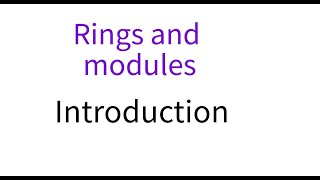Rings and modules 1 Introduction

This lecture is part of an online course on ring theory, at about the level of a first year graduate course or honors undergraduate course. This is the introductory lecture, where we recall some basic definitions and examples, and describe the analogy between groups and rings. For the

From playlist Rings and modules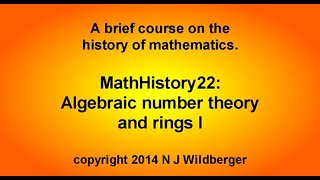Algebraic number theory and rings I | Math History | NJ Wildberger

In the 19th century, algebraists started to look at extension fields of the rational numbers as new domains for doing arithmetic. In this way the notion of an abstract ring was born, through the more concrete examples of rings of algebraic integers in number fields. Key examples include

From playlist MathHistory: A course in the History of Mathematics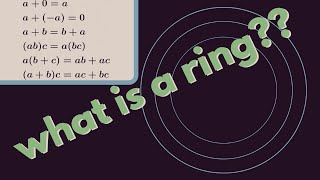Abstract Algebra | What is a ring?

We give the definition of a ring and present some examples. http://www.michael-penn.net http://www.randolphcollege.edu/mathematics/

From playlist Abstract Algebra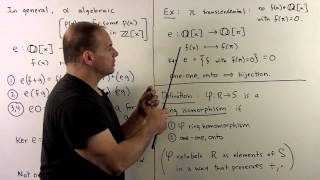RNT1.3. Ring Homomorphisms

Ring Theory: We define ring homomorphisms, ring isomorphisms, and kernels. These will be used to draw an analogue to the connections in group theory between group homomorphisms, normal subgroups, and quotient groups.

From playlist Abstract Algebra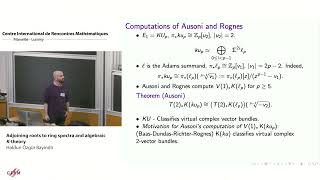Haldun Özgür Bayindir : Adjoining roots to ring spectra and algebraic 𝐾-theory

CONFERENCE Recording during the thematic meeting : « Chromatic Homotopy, K-Theory and Functors» the January 24, 2023 at the Centre International de Rencontres Mathématiques (Marseille, France) Filmmaker: Jean Petit Find this video and other talks given by worldwide mathematicians on CIR

From playlist Topology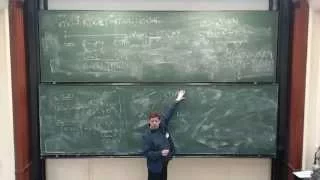Charles Rezk: Elliptic cohomology and elliptic curves (Part 1)

The lecture was held within the framework of the Felix Klein Lectures at Hausdorff Center for Mathematics on the 1. June 2015

From playlist HIM Lectures 2015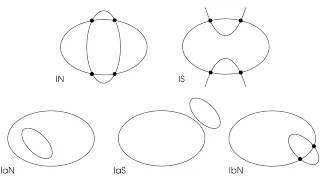"New Paradigms in Invariant Theory" - Roger Howe, Yale University 

HKUST Institute for Advanced Study Distinguished Lecture New Paradigms in Invariant Theory Speaker: Prof Roger Howe, Yale University Date: 13/6/2011 Video taken from: http://video.ust.hk/Watch.aspx?Video=6A41D5F6B1A790DC

From playlist Mathematics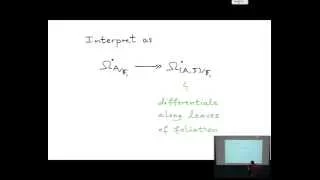Lars Hesselholt: The big de Rham Witt complex

The lecture was held within the framework of the Hausdorff Trimester Program: Non-commutative Geometry and its Applications and the Workshop: Number theory and non-commutative geometry 26.11.2014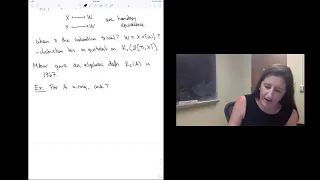Teena Gerhardt - 1/3 Algebraic K-theory and Trace Methods

Algebraic K-theory is an invariant of rings and ring spectra which illustrates a fascinating interplay between algebra and topology. Defined using topological tools, this invariant has important applications to algebraic geometry, number theory, and geometric topology. One fruitful approacTour of My Abstract Algebra Book Collection

In this video I go over some of my abstract algebra books. I am pretty sure I have more but I am not 100% positive. I have more bookshelves and boxes I need to go through still, I have a lot of books!! There are a lot of books here. Keep in mind this collection has taken me years. If you

From playlist Book Reviews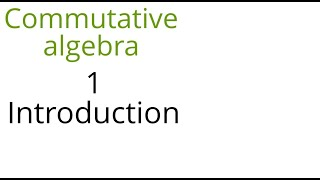Commutative algebra 1 (Introduction)

This lecture is part of an online course on commutative algebra, following the book "Commutative algebra with a view toward algebraic geometry" by David Eisenbud. https://link.springer.com/book/10.1007/978-1-4612-5350-1 This is a short introductory lecture, and gives a few examples of the

From playlist Commutative algebra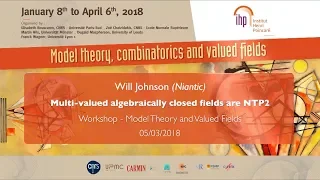Multi-valued algebraically closed fields are NTP₂ - W. Johnson - Workshop 2 - CEB T1 2018

Will Johnson (Niantic) / 05.03.2018 Multi-valued algebraically closed fields are NTP₂. Consider the expansion of an algebraically closed field K by 𝑛 arbitrary valuation rings (encoded as unary predicates). We show that the resulting structure does not have the second tree property, and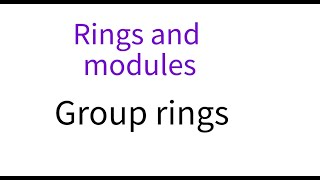Rings and modules 2: Group rings

This lecture is part of an online course on rings and modules. We decribe some examples of rings constructed from groups and monoids, such as group rings and rings of Dirichlet polynomials. For the other lectures in the course see https://www.youtube.com/playlist?list=PL8yHsr3EFj52XDLrm

From playlist Rings and modules